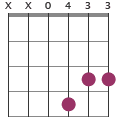G chord inversions

G/B and G/D are the first and second inversions of the G major. This means that the bass tone is shifting from G to B or D. Both these chords are also referred to as slash chords.

G/B

•G/D

•Try in a chord progression

C - G/B - Am
Em - G/D - D

Theory of the G/B and G/D chords

Both chords consist of the notes G, B, D. The difference is that the order of notes shifts. B is the bass note in the first inversion and D is the bass note in the second inversion.

A comparison between the main G major and the two inversions can be seen below.

Chord construction

G  G - B - D - G - B - G
G/B  x - B - D - G - B - G
G/D  x - x - D - B - D - G
Guitar versions of the chord

Notes in chord

G  G - B - D
G/B  B - D - G
G/D  D - G - B
Only the order of notes differs